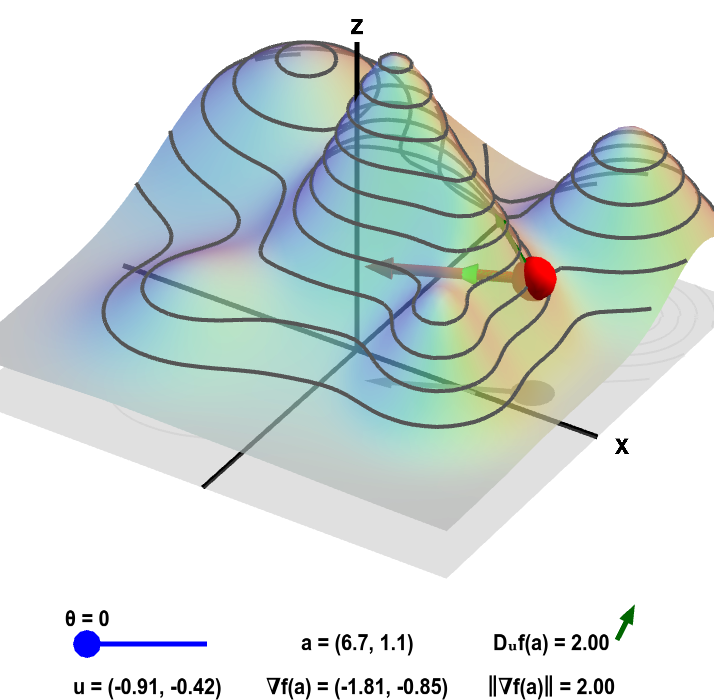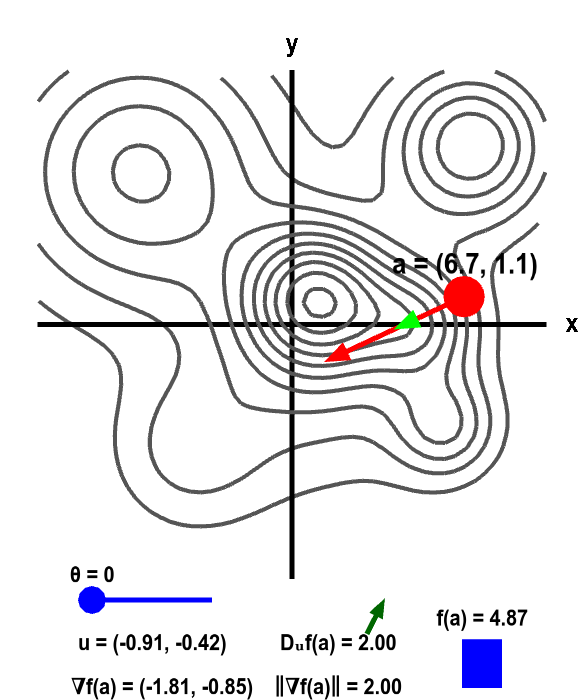# Math Insight

### Applet: Gradient and directional derivative on a mountainThe height of a mountain range described by a function $f(x,y)$ is shown as surface plot in three-dimensions (left) and a two-dimensional level curve plot (right). In each panel, a red point can be moved by the mouse to change the location $\vc{a}$ where the gradient $\nabla f(\vc{a})$ is calculated. Since $f$ is a function of two variables, the point $\vc{a}$ and the gradient are two-dimensional. The two-dimensional point $\vc{a}$ is illustrated by the shadow of the red point on the $xy$-plane below the surface plot and by the red point itself on the level curve plot. The two dimensional gradient vector $\nabla f(\vc{a})$ is illustrated by the red vector emanating from the red point as well as by its shadow below the surface plot.

The light green two-dimensional vector $\vc{u}$ points at an angle $\theta$ (changeable via the slider) from the gradient. (It is also represented by its shadow below the surface plot.) The value of the directional derivative $D_{\vc{u}}f(\vc{a})$ is the slope of the dark green vector tangent to the surface, which is reproduced next to the value of $D_{\vc{u}}f(\vc{a})$ shown at the bottom of each panel. The value of the directional derivative is compared to the magnitude of the gradient $\| \nabla f(\vc{a})\|$. The height of the surface $f(\vc{a})$ is also illustrated by the bar in the lower right of the second panel.